Courses

# Test: Matrices- 1

## 25 Questions MCQ Test Mathematics For JEE | Test: Matrices- 1

Description
This mock test of Test: Matrices- 1 for JEE helps you for every JEE entrance exam. This contains 25 Multiple Choice Questions for JEE Test: Matrices- 1 (mcq) to study with solutions a complete question bank. The solved questions answers in this Test: Matrices- 1 quiz give you a good mix of easy questions and tough questions. JEE students definitely take this Test: Matrices- 1 exercise for a better result in the exam. You can find other Test: Matrices- 1 extra questions, long questions & short questions for JEE on EduRev as well by searching above.
QUESTION: 1

### The number of all possible matrices of order 3×3 with each entry 0 if 1 is

Solution:

23x3 = 29 = 512.

The number of elements in a 3 X 3 matrix is the product 3 X 3=9.

Each element can either be a 0 or a 1.

Given this, the total possible matrices that can be selected is 29=512

QUESTION: 2

###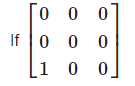Solution:

If any row or column of a square matrix is 0 , then its product with itself is always a zero matrix.

QUESTION: 3

### This matrix is a _______ .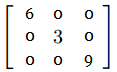Solution:
QUESTION: 4

Two matrices A and B are multiplicative inverse of each other only if

Solution:

If AB = BA = I , then A and B are inverse of each other. i.e. A is invers of B and B is inverse of A.

QUESTION: 5

For what value of λ the following system of equations does not have a solution ? x + y + z = 6, 4x + λy - λz = 0, 3 x + 2y – 4 z = - 5

Solution:

The given system of equations does not have solution if :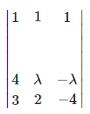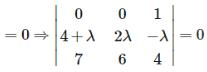⇒ (24 + 6λ - 14λ) = 0 ⇒ λ = 3

QUESTION: 6

I2 is the matrix

Solution: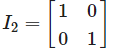In linear algebra, the identity matrix, or sometimes ambiguously called a unit matrix, of size n is the n × n square matrix with ones on the main diagonal and zeros elsewhere. It is denoted by In, or simply by I if the size is immaterial or can be trivially determined by the context

QUESTION: 7

Let A be any m×n matrix, then A2 can be found only when

Solution:

The product of any matrix with itself can be found only when it is a square matrix.i.e. m = n.

QUESTION: 8

The order of the single matrix obtained from is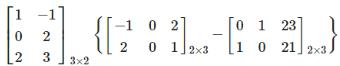Solution: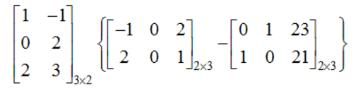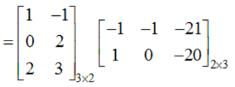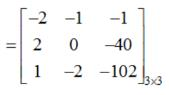QUESTION: 9

If A and B are square matrices of the same order, then(A+B)2 = A2+2AB+B2 implies

Solution:

If A and B are square matrices of same order , then , product of the matrices is not commutative.Therefore , the given result is true only when AB = BA.

QUESTION: 10

The value of λ, for which system of equations. x + y + z = 1, x + 2y + 2z = 3, x + 2y + λz = 4, have no solution is

Solution:

The given system of equations does not have solution if :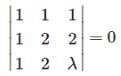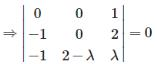⇒ (-2 + λ) = 0 ⇒ λ = 2

QUESTION: 11

If A is a matrix of order 3 × 4 , then each row of A has

Solution: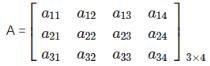, therefore matrix A has 4 elements in each row

QUESTION: 12

If A and B are any two matrices, then

Solution:

Let matrix A is of order m x n , and matrix B is of order p x q . then , the product AB is defined only when n = p. that’s why, If A and B are any two matrices, then AB may or may not be defined.

QUESTION: 13

If, we are given a square matrix A then, Adj.(KA) = ….

Solution:

Adj.(KA) = Kn−1 Adj.A , where K is a scalar and A is a n x n matrix.

QUESTION: 14

Let A =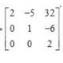, then adj A is

Solution: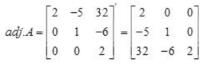Explanation :  A = {(1,0,0) (5,2,0) (-1,6,1)}

a11 = 2, a12 = -5, a13 = 32

a21 = 0, a22 = 1, a23 = -6

a31 = 0, a32 = 0, a33 = 2

then A becomes = {(2,-5,32) (0,1,-6) (0,0,2)}

QUESTION: 15

The system of equations,x + y = 2 and 2x + 2y = 3 has

Solution:

For No solutions,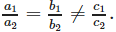for given system of equations we have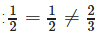QUESTION: 16

If P is of order 2 × 3 and Q is of order 3 × 2, then PQ is of order

Solution:

Here, matrix P is of order 2 × 3 and matrix Q is of order 2 × 2 , then , the product PQ is defined only when : no. of columns in P = no. of rows in Q. And the order of resulting matrix is given by : rows in P x columns in Q.

QUESTION: 17

A square matrix A = [aij]n×n is called a lower triangular matrix if aij = 0 for

Solution:

Lower triangular matrix is given by :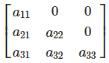,

here , aij = 0
if i is less than j.and aij ≠ 0, if i is greater than j.

QUESTION: 18

If A and B are any two square matrices of the same order, then

Solution:

By the property of transpose , (AB)’ = B’A’.

QUESTION: 19

The transformation ‘orthogonal projection on X-axis’ is given by the matrix

Solution:

The orthogonal projection on x- axis is given by :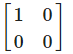QUESTION: 20

The equations x + 2y + 2z = 1 and 2x + 4 y + 4z = 9 have

Solution:

The given system of equations does not have solution if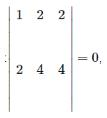QUESTION: 21

The number of all the possible matrices of order 2 × 2 with each entry 0, 1 or 2 is

Solution:

32x2 = 34 = 81

QUESTION: 22

A square matrix  A = [aij]n×n is called an upper triangular if aij = 0 for

Solution:

Upper Triangular matrix is given by  :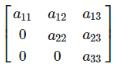.
Here, aij=0 , if i is greater than j.and aij ≠ 0, if I is less than j.

QUESTION: 23

If A is any square matrix, then

Solution:

For every square matrix (A + A’) is always symmetric.

QUESTION: 24

The equations, x + 4 y – 2 z = 3, 3 x + y + 5 z = 7, 2 x + 3y +z = 5 have

Solution:

The given system of equations does not have solution if :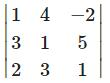- 0 ⇒ 1(-14) - 4(-7) -2(7) = 0

QUESTION: 25

If the system of equationsx + 4 ay + az = 0, x + 3by + bz = 0 andx + 2 cy +cz = 0 have a non-zero solution,then a, b, c are in

Solution:

For a non trivial solution :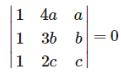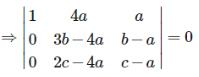⇒ bc + ab - 2ac = 0 ⇒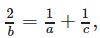∴ there, a , b ,c, are in H.P